# Statistical test

(diff) ← Older revision | Latest revision (diff) | Newer revision → (diff)

A decision rule according to which a decision is taken in the problem of statistical hypotheses testing (cf. Statistical hypotheses, verification of) on the basis of results of observations.

Assume that the hypothesis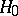:has to be tested against the alternative: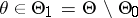by means of the realization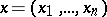of a random vector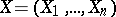that takes values in a sample space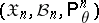,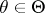. Furthermore, letbe an arbitrary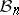-measurable function, mapping the sample space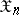onto the interval. In a case like this, the principle according to whichis rejected with probability, while the alternative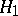is rejected with probability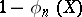, is called a statistical test for testingagainst;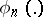is the critical function of the test. The function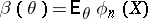,, is called the power function of the test.

The use of a statistical test leads either to a correct decision being taken, or to one of the following two errors being made: rejection of, and thus acceptance of, when in factis correct (an error of the first kind), or acceptance ofwhen in factis correct (an error of the second kind). One of the basic problems in the classical theory of statistical hypotheses testing is the construction of a test that, given a definite upper bound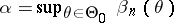,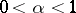, for the probability of an error of the first kind, would minimize the probability of an error of the second kind. The numberis called the significance level of the statistical test.

In practice, the most important are non-randomized statistical tests, i.e. those with as critical functionthe indicator function of a certain-measurable setin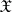: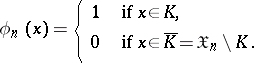Thus, a non-randomized statistical test rejectsif the eventtakes place; on the other hand, if the event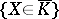takes place, thenis accepted. The setis called the critical region of the statistical test.

As a rule, a non-randomized statistical test is based on a certain statistic, which is called the test statistic, and the critical regionof this same test is usually defined using relations of the form,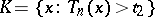,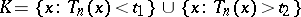. The constants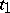,, called the critical values of the test statistic, are defined from the condition; in these circumstances one speaks in the first two cases of one-sided statistical tests, and in the third case, of a two-sided statistical test. The structure of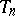reflects the particular nature of the competing hypothesesand. In the case where the familypossesses a sufficient statistic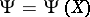, it is natural to look for the test statistic in the class of sufficient statistics, sincefor all, where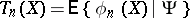.

How to Cite This Entry:
Statistical test. Encyclopedia of Mathematics. URL: http://encyclopediaofmath.org/index.php?title=Statistical_test&oldid=12586
This article was adapted from an original article by M.S. Nikulin (originator), which appeared in Encyclopedia of Mathematics - ISBN 1402006098. See original article